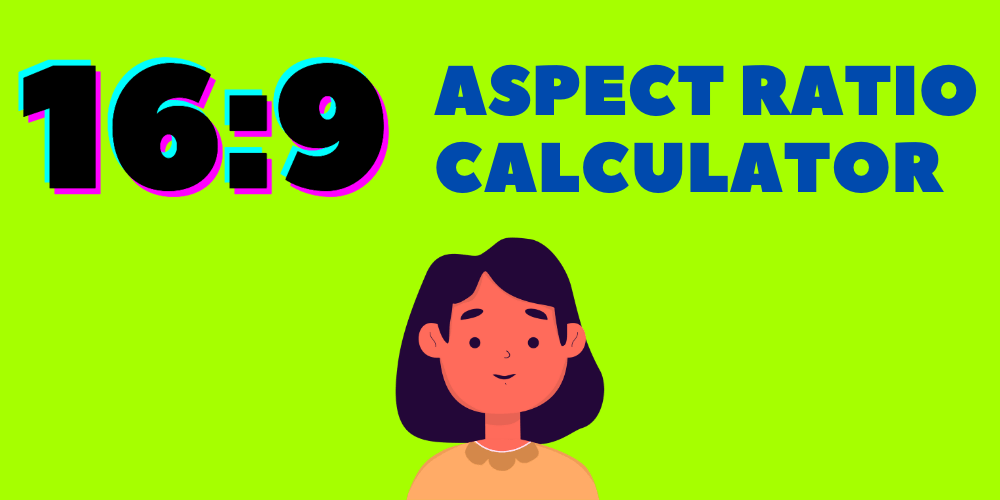# 16:9 Aspect Ratio CalculatorAspect Ratio Calculator

The actual height needed to maintain a 16:9 aspect ratio is:

The final dimensions:

Are you tired of trying to calculate the perfect aspect ratio for your project, only to end up with subpar results? Look no further, because we have developed the ultimate 16:9 aspect ratio calculator to help you achieve the perfect dimensions every time.

Our calculator takes into account a variety of factors, including the original aspect ratio, the desired final aspect ratio, and the resizing method. Whether you want to fit your content within a specific frame size or crop excess pixels, our calculator has got you covered.

But that’s not all – we also provide in-depth explanations and visual examples to help you better understand the calculations and the resulting dimensions. So not only will you get the right numbers, but you’ll also have a deeper understanding of how aspect ratios work.

## How to Use Our 16:9 Aspect Ratio Calculator

Using our calculator is simple – just follow these steps:

1. Enter your desired width in the given box. Just the number will do. Or bring your cursor over to the right side of the box, you will see up and down arrows. Click on the arrow pointing up to bring up the values.
2. You will see the height generated in the next line.
3. The final dimensions will also be shown in the form of width x height.

Here’s an example of how the calculator might be used:

Original Aspect Ratio: 4:3
Final Aspect Ratio: 16:9
Resizing Method: Fit within frame

Result: The resulting dimensions for your content will be 960 pixels wide and 540 pixels tall.

You can do the same for 4:3 aspect ratio as well by using the width to find the height and final dimension.

## Understanding the Calculations

So how does our calculator come up with these numbers? Let’s take a closer look at the math behind it.

### Fit Within Frame

When the “fit within frame” option is selected, the calculator adjusts the content so that it fits within the desired aspect ratio while maintaining the original aspect ratio’s proportions. This means that some of the content may be cropped in order to fit the frame.

To calculate the dimensions, we first find the difference between the original and final aspect ratios by dividing the final aspect ratio’s width by the original aspect ratio’s width, and the final aspect ratio’s height by the original aspect ratio’s height.

For example, if the original aspect ratio is 4:3 and the final aspect ratio is 16:9, the differences would be:

Final aspect ratio width / Original aspect ratio width = 16 / 4 = 4
Final aspect ratio height / Original aspect ratio height = 9 / 3 = 3

Next, we take the smaller of the two differences and multiply it by the original aspect ratio’s dimensions. In this case, 3 is the smaller difference, so we multiply the original aspect ratio’s dimensions (4 and 3) by 3 to get the resulting dimensions:

Width: 4 * 3 = 12
Height: 3 * 3 = 9

### Expand to Fit Frame

The “expand to fit frame” option works in a similar way to “fit within frame,” but it adjusts the content so that it fills the entire frame while maintaining the original aspect ratio’s proportions. This means that some of the frame

## Interactive Diagram

To help visualize the different resizing methods, we’ve included an interactive diagram below. Simply click on the boxes to see how the content would look when fit within the frame, expanded to fill the frame, or cropped to fit the frame.

``````graph LR
A[Original Aspect Ratio] --> B(Fit Within Frame)
A --> C(Expand to Fit Frame)
A --> D(Crop Excess Pixels)
B --> E[Resulting Dimensions]
C --> E
D --> E
``````

## Conclusion

We hope that our 16:9 aspect ratio calculator has made it easier for you to achieve the perfect dimensions for your content. Whether you’re looking to fit within a specific frame size or expand to fill the entire frame, our calculator has got you covered. And with the in-depth explanations and visual examples, you’ll not only get the right numbers, but you’ll also have a deeper understanding of how aspect ratios work.

So next time you’re struggling to calculate the perfect aspect ratio, remember to give our calculator a try. You won’t be disappointed.